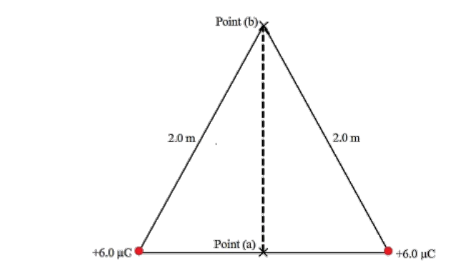Problem: Two-point charges +9.0 μC are affixed at the corners of the base of an equilateral triangle, as shown in the figure. A third charge +3.3 μC is first placed midway between two charges at point (a). Find the work done by the two charges as it moves from point (a) to (b). (k = 1/4πε0 = 8.99 x 109 N•m2/C2).

FREE Expert Solution
92% (272 ratings)
Problem Details

Two-point charges +9.0 μC are affixed at the corners of the base of an equilateral triangle, as shown in the figure. A third charge +3.3 μC is first placed midway between two charges at point (a). Find the work done by the two charges as it moves from point (a) to (b). (k = 1/4πε0 = 8.99 x 109 N•m2/C2).